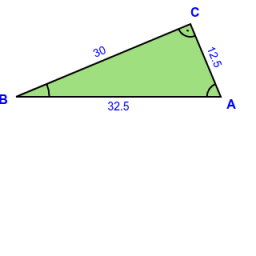# Perpendicular 70824

One perpendicular to the right triangle ABC has a length a = 14 cm, and a radius of the circle inscribed in this triangle r = 5 cm. Find the size of the diaphragm and its second perpendicular.

c =  26.5 cm
b =  22.5 cm

### Step-by-step explanation:

Try calculation via our triangle calculator.Did you find an error or inaccuracy? Feel free to write us. Thank you!

Tips for related online calculators
Need help calculating sum, simplifying, or multiplying fractions? Try our fraction calculator.
The Pythagorean theorem is the base for the right triangle calculator.
Do you want to convert time units like minutes to seconds?

#### Grade of the word problem:

We encourage you to watch this tutorial video on this math problem: